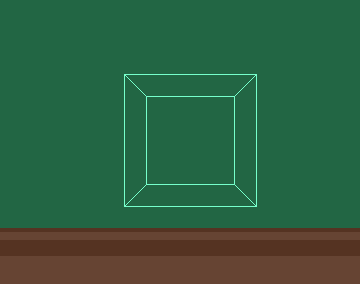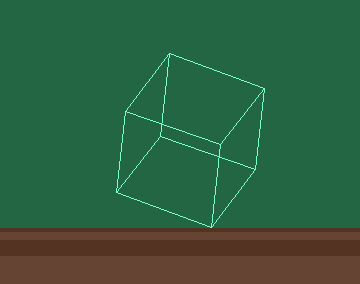# 4. The Year of the Megademo

Developing Demo Effects #4
It is the year 1989. This is the year in which the megademo became a true phenomenon, with major groups proving their professionalism and talent in getting a megademo produced. And some of those megademos started featuring 3D effects, such as the classic Red Sector Megademo.
Megademos consist of standalone demos, where each enclave of members (artists, musicians, coders) have full creative control over their own demo. The demos are then put on one or more disks, thus becoming parts of the megademo, together with a loader or loader part to navigate between them.

3D effects had a magic all its own from the start. Here's this humble home computer with a quite modest CPU and a 2D low-resolution screen of pixels, and yet, on the screen is a simulation of a spaceship navigating through space in real-time!
Luckily, the Amiga had hardware accelerated line drawing. In 1989, there was a big push for getting this magic running smoothly. Lots of coders learned the hardware independently. But before they could take the hardware for a spin, they had to learn how to rotate..!

## 3D vector calculations

The following is a summary of a simple rotation of a single object. For complete 3D scenes, matrix transforms are more generally versatile.
3D objects, as the name suggests, live in three dimensions of space: X, Y, Z, describing the horizontal, vertical, and depth position of each corner, or vertex, that make up objects in this space. The screen is flat and has only two dimensions, so to give the illusion of depth, the positions (vertices) must be recalculated.
Consider a cube, seen from the front in isometric 3D (=no perspective):Figure 1a: A 3D cube in coordinate space. The back (far Z) vertices have the same X and Y coordinates, and are exactly behind the front vertices in isometric 3D from this viewpoint.
We can scale by Z to get perspective, as we did in Developing Demo Effects #2 - the Chessboard effect:Figure 1b: The same 3D cube after applying perspective calculations.
In our effect, we rotate the object in 3D space:Figure 2a: First, the object is rotated in 3D space, and can be represented isometrically.
...and then scale the X and Y coordinates by Z. That's it!Figure 2b: Then, perspective calculations are applied to create the illusion of depth and also to adapt the coordinate values to the screen size.

As you may have guessed, to make a vector bobs cube, we just place bobs at each point (vertex) instead of drawing lines between them.Figure 3: The same method is used to place bobs at the vertices instead. Bob graphic by Vincent Bijwaard a.k.a Alien of Paradox
To write a 3D rotation function is non-trivial. But if we break it down into steps, it might be easier to intuit, provided you come armed with some geometry. :)

## 2D rotation

Take the simpler case of rotation around a single axis, for example the Z axis. The X,Y point is a point somewhere along the periphery of a circle, and can therefore be described as sums of expressions on the form of x=r*cos(az) or y=r*sin(az) (where az is the angle around the Z axis):
```x1 = x0*cos(az) - y0*sin(az)
y1 = x0*sin(az) + y0*cos(az)
```Figure 4: The simpler 2-D rotation.
For the cos and sin functions we will use a sine wave to look up values from, as for the bob snake in the previous article, but the amplitude will be 32767 - adapted to maximized calculation precision.

## 3D rotation

You can certainly write long equations to solve x,y,z for a rotation, but a common way is to rotate around one axis and take the rotated point as the point for the next rotation, i.e. calculate a partial result used as input for the next calculation step. Here, it is important to keep the partial results within the used coordinate space (calculation precision).
Note that just because we've divided the rotation into steps for convenience, this does not mean that the rotation is sequential in nature. We would like to rotate the object around its own axes, but that requires quaternion math. The rotation axes in a normal rotation transform are not the object's axes, but the axes of the coordinate space in which we rotate it.

```x1 = x0*cos(az) - y0*sin(az)
y1 = x0*sin(az) + y0*cos(az)
z1 = z0

y2 = y1*cos(ax) - z1*sin(ax)
z2 = y1*sin(ax) + z1*cos(ax)
x2 = x1

z3 = z2*cos(ay) - x2*sin(ay)
x3 = z2*sin(ay) + x2*cos(ay)
y3 = y2
```

Where x0, y0, z0 is the 3D point in the 3D object's space, and x3, y3, z3 is the rotated object's coordinates in world space. This world space is then mapped onto the screen, oriented so that the Z axis points out of the screen.
These equations together represent the cross product of a vector and a transformation matrix. (A special-case matrix with only rotation - no scaling or translation. The word 'vector' used here means the mathematical term, a (3D) point.)

With two macros for scaling by the sine or cosine value, the code becomes quite short:
```SinScale:MACRO
muls (a5,\1.w),\2
swap \2
ENDM

CosScale:MACRO
muls (a6,\1.w),\2
swap \2
ENDM

...
*--- rotation az ---*		;d1-d3 = x0,y0,z0
move.w d1,d4			;x1 = x0*cos(az) - y0*sin(az)
CosScale a4,d4
move.w d2,d5
SinScale a4,d5
sub.w d5,d4			;d4=x1

move.w d1,d5			;y1 = x0*sin(az) + y0*cos(az)
SinScale a4,d5
CosScale a4,d2
add.w d5,d2			;d2=y1; z1 = z0 = d3 = nop :)
*--- rotation ax ---*
move.w d2,d5			;y2 = y1*cos(ax) - z1*sin(ax)
CosScale a2,d5
move.w d3,d6
SinScale a2,d6
sub.w d6,d5			;d5=y2

move.w d2,d6			;z2 = y1*sin(ax) + z1*cos(ax)
SinScale a2,d6
CosScale a2,d3
add.w d6,d3			;d3=z2; x2 = x1 = d4 = nop :)
*--- rotation ay ---*
move.w d3,d6			;z3 = z2*cos(ay) - x2*sin(ay)
CosScale a3,d6
move.w d4,d7
SinScale a3,d7
sub.w d7,d6			;d6=z3

SinScale a3,d3			;x3 = z2*sin(ay) + x2*cos(ay)
CosScale a3,d4
add.w d3,d4			;d4=x3; y3 = y2 = d5 = nop :)
*--- done ---*			;d4,d5,d6 = x3,y3,z3
...
```

## Perspective calculation

The screen is of course flat, or 2D. Therefore, the x3 and y3 coordinates are divided by z3 to shrink the object with distance. This is what creates the illusion of perspective, by projecting it onto the screen.
Dividing x3 and y3 by z3 would always result in a number between -1 and 1. In integer arithmetic, this is obviously too small, and also, 2px objects are not very interesting or impressive! So z3 is first scaled down to leave the divided x3,y3 coordinates at a decent size in pixels, and an eye distance is added. This, together, determines the FOV, or Field of View.
```PERSP2D:MACRO			;final projection from world to screen coords
ext.l d4
ext.l d5
neg.w d6		;world +z is forward,screen perspective is away
asr.w #8,d6		;this scaling
add.w #78,d6		;and eye Z determines Field of View.
divs d6,d4
divs d6,d5
add.w #w/2,d4		;center horizontally on the screen
ENDM
```

## Writing the 3D calculation routine

For the 3D calculation routine to be as flexible and universal as possible, it should read a list of 3D coordinates and output a list of perspective-projected 2D coordinates scaled to screen size.
To define something to be shown, we use large values for the coordinates. This is the "3D world" scale; in our case, we've defined a simple object so that it's 1/8th of the total world size (about 8x8 screens in size). To this is added a line list, which describes how to "connect the dots". It contains pairs of coordinate offsets (into the coordinate list) for the start and end point of each line; the coordinates are numbered from 0 upward.
```max	=4095				;+x,y,z rightward, upward, forward
max2	=max*2/3

VertexCount:
dc.w MaxVerts
Vertices:
dc.w max,max,max		;back surface, counter-clockwise
dc.w -max,max,max
dc.w -max,-max,max
dc.w max,-max,max

dc.w max2,max2,0		;middle ring, counter-clockwise
dc.w -max2,max2,0
dc.w -max2,-max2,0
dc.w max2,-max2,0

dc.w max,max,-max		;front surface, counter-clockwise
dc.w -max,max,-max
dc.w -max,-max,-max
dc.w max,-max,-max

Lines:
dc.w 0*6,1*6, 1*6,2*6, 2*6,3*6, 3*6, 0*6	;back surface
dc.w 0*6,4*6, 1*6,5*6, 2*6,6*6, 3*6, 7*6	;connect corners to...
dc.w 4*6,5*6, 5*6,6*6, 6*6,7*6, 7*6, 4*6	;middle ring
dc.w 4*6,8*6, 5*6,9*6, 6*6,10*6, 7*6, 11*6	;connect corners to...
dc.w 8*6,9*6, 9*6,10*6, 10*6,11*6, 11*6, 8*6	;front surface
```

## Drawing bobs

Making vector bobs simply constitutes blitting a bob at the 2D point that we calculated from each vertex. Our previous bob example source blits bobs fine, so it's just a case of adding the 3D calculations to that.
In our case, all the bobs are the same size, and a fixed x,y offset is subtracted to put the center (instead of the upper left corner) of the bob at the specified (x,y) coordinate. But if you use bobs of mixed size, you must store the center point offset to subtract for each bob size.
There is one more thing you must do (unless all bobs are a single color) - sort the 2D coordinates by its Z coordinate. I've written a simple sort that is relatively efficient for typical coordinate counts, to illustrate:

## Swapless Reference Sort

```SwaplessReferenceSort:			;d0/a0-a1=count-1,Coords,Coordptrs
move.w d0,d2			;count-1
.sortl:					;outer loop to build output
move.w d2,d1			;count-1
move.l a0,a2			;go to start of array
bra.s .newmin
.scanl:					;inner loop to scan for minimum
cmp.w (a2),d3			;new minimum value?
ble.s .nomin
.newmin:move.w (a2),d3			;set minimum
move.l a2,a3			;and its pointer (reference)
dbf d1,.scanl

add.w #\$4000,(a3)		;flag value as handled (put above max)
move.l a3,(a1)+			;minptr stored
dbf d0,.sortl
rts
```

Effect improvements include animation / morphing, shading or scaling bobs by distance, etc.

## Drawing lines

On the Amiga, the Blitter has a line drawing mode that gives great performance enhancements. This mode is completely different from normal mode. Bits in BLTCON1 are set according to the angle of the line, and you put delta values in some of the well-known registers normally used for other things. The Blitter registers are then interpreted differently from their normal contents, when this mode is enabled.
In order for a line-draw routine to be as flexible and useful as possible, it should be abstracted from any notion of 3D and just draw a line from one screen coordinate to another:
```LineDraw:				;d0-d4/a1=x1,y1,x2,y2,bwid,screen ptr. a3/a4=\$42,\$bfa0000f
ror.l #4,d6			;shift it in at top, low word cleared.

*--- deltas ---*

sub.w d1,d3
bpl.s .dyplus
neg.w d3
.dyplus:
sub.w d0,d2
bgt.s .dxplus
neg.w d2
.dxplus:
cmp.w d2,d3
bge.s .dylarger
exg d2,d3			;d2=Small delta, d3=Large delta
.dylarger:

*--- blit values ---*

muls d4,d1			;line offset=y*bwid
asr.w #3,d0			;bit 0 ignored by Blitter

move.w d2,d5			;2*SDelta
swap d2
sub.w d3,d5
smi d0				;if (2*Sdelta-Ldelta) < 0,
sub.b d0,d6			;add 1 (subtract -1) to octant lookup.
move.b OctTbl(PC,d6.w),d6	;look up BLTCON bits for octant

move.w d5,d2			;2*Sdelta-Ldelta
sub.w d3,d2			;2*Sdelta-2*Ldelta
asl.w #6,d3

*--- blit ---*

WAITBLIT
move.l d6,BLTCON0(a6)		;shift, minterm, octant bits
move.w d5,BLTAPTL(a6)		;2*Sdelta-Ldelta
move.l d2,BLTBMOD(a6)		;2*Sdelta | 2*Sdelta-2*Ldelta
move.l d1,BLTCPTH(a6)		;source
move.l d1,BLTDPTH(a6)		;destination
move.w d3,BLTSIZE(a6)
rts
```

It is then adapted to our specific effect with a loop that reads the coordinates and calls LineDraw:
```DrawObj:				;d7/a0-a2=count-1,Lines,screen,Coords
moveq #-1,d0			;A mask (always -1)
move.w #\$8000,d1		;line width 1px (others look bad)
move.w #\$ffff,d2		;line pattern (word)
move.w #bwid,d4			;screen width in bytes
bsr LineInit			;Initialize Blitter and CPU registers
.linel:
move.w (a0)+,d0
movem.w (a2,d0.w),d0-d1
move.w (a0)+,d2
movem.w (a2,d2.w),d2-d3
bsr LineDraw
dbf d7,.linel
rts
```

Effect improvements include hidden face removal, object animation / vertex morphing, object subdivision / line curvature, 3D clipping, and much more.

## Inspiration!

Now bring out the classic grid paper or write a 3D object converter, and make some nice objects! Maybe you can experiment and find more ways to make them more visually interesting and impressive?